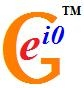# User blogs

Tag search results for: "continuous"Scales Solve the Continuous vs. Discrete Paradox (by Ray B. Munroe, Jr.): Abstract: It is the author’s position that Nature is fundamentally both continuous and discrete, and that this paradox is directly responsible for the wave-particle duality of Nature. Two key catalysts in the collapse of continuous wave functions (waves) into sets of discrete quantum numbers (particles) are 1) Scales and 2) Lucas Numbers. These concepts – along with Supersymmetry – may provide the framework for the ultimate unification of bosons and fermions. http://prespacetime.com/index.php/pst/article/view/201

Mathematical Emptiness: The Illusion-like Effectiveness of Mathematical Mind (by Graham P. Smetham): Abstract: The view of the interdependent genesis of the two realms of dualistic manifestation; the realm of ‘physicality’, which is the objective aspect of the dualistic manifestation from the deeper, unitary, implicate (to use a term for levels of non-duality used by physicist David Bohm) dimension of Mindnature, and the subjective realm of individuated ‘mentality’ solves a crucial puzzle that has bothered many physicists and mathematicians. Eugene Wigner, for instance, referred to what he considered to be the ‘unreasonable effectiveness’ of mathematics in describing and explaining the physical world of ‘nature’; he called mathematics a ‘miracle’ and ‘a wonderful gift that we neither understand nor deserve.’ However, if both the realm of mentality and physicality emerge from a deeper level of universal Mindnature then it is surely not such a great mystery that mathematics, itself a product of mind, produces the conceptual patterns generated and followed by the ‘physical’ functioning of reality. http://prespacetime.com/index.php/pst/article/view/202

Infinite Resolution (by Cristinel Stoica): Abstract: It was reported that General Relativity predicts its own breakdown, because of singularities. We will see that the mathematics of General Relativity can be naturally extended to work fine when the metric becomes degenerate. Then is proposed an extended version of Einstein's equation which remains valid at singularities. The time evolution is expressed by equations which allow passing beyond the singularities. Consequently, the problems of singularities, including Hawking's information paradox, vanish. The core principle used to extend the mathematics and physics of General Relativity beyond the singularities provides a surprising answer to the question: Is there a deep, foundational reason why reality must be purely analog, or why it must be digital?" http://prespacetime.com/index.php/pst/article/view/203

On the Z=(t/z)-Type Solutions of Plane Gravitational Waves of Non-Symmetric Unified Field Equations of Einstein, Bonnor and Schrodinger in a Generalized Takeno Space-time (by Sanjay R. Bhoyar, R. S. Rane, S. D. Katore, A. G. Deshmukh): Abstract: In this paper, wave solutions of both the weak and strong, non-symmetric unified field equations of Einstein, Bonner and Schrodinger have been investigated in a generalized Takeno space-time deduced by Bhoyar and Deshmukh  for Z= (t/z)-type plane gravitational waves. It has been shown that the plane wave solutions Einstein and Bonner equations exists but Schrodinger field equations have no solutions in this space-time. http://prespacetime.com/index.php/pst/article/view/195

An Inhomogeneous Cosmological Model in Electromagnetic Bulk Viscous Fluid with Variable (Λ) (by Sharad P. Kandalkar, Pramod P. Khade, Sunita P. Gawande): Abstract: An inhomogeneous cylindrically symmetric cosmological model for bulk viscous fluid distribution with electromagnetic field is obtained. To get a deterministic solution, it is assumed that the component σ11 of shear tensor σji is proportional to expansion ɵ and the coefficient of bulk viscosity is assumed to be a power function of mass density. The source of the magnetic field is due to an electric current produced along z-axis. Some physical and kinematical aspects of the models are also discussed in presence and absence of the magnetic field. http://prespacetime.com/index.php/pst/article/view/197

· Jul 12 '12 ·Discrete Time and Kleinian Structures in Duality between Spacetime and Particle Physics (by Lawrence B. Crowell): Abstract: The interplay between continuous and discrete structures results in a duality between the moduli space for black hole types and AdS7 spacetime. The 3 and 4 Q-bit structures of quantum black holes is equivalent to the conformal completion of AdS. http://prespacetime.com/index.php/pst/article/view/198

In Defense of Octonions (by Jonathan J. Dickau, Ray B. Munroe, Jr.): Abstract: Various authors have observed that the unit of the imaginary numbers, i, has a special significance as a quantity whose existence predates our discovery of it. It gives us the ability to treat degrees of freedom in the same way mathematically that we treat degrees of fixity. Thus; we can go beyond the Real number system to create or describe Complex numbers, which have a real part and an imaginary part. This allows us to simultaneously represent quantities like tension and stiffness with real numbers and aspects of vibration or variation with imaginary numbers, and thus to model something like a vibrating guitar string or other oscillatory systems. But if we take away the constraint of commutativity, this allows us to add more degrees of freedom, and to construct Quaternions, and if we remove the constraint of associativity, what results are called Octonions. We might have called them super-Complex and hyper-Complex numbers. But we can go no further, to envision a yet more complicated numbering system without losing essential algebraic properties. A recent Scientific American article by John Baez and John Huerta suggests that Octonions provide a basis for the extra dimensions required by String Theory and are generally useful for Physics, but others disagree. We examine this matter.

Nonlinear Theory of Elementary Particles Part XI: On the Structure and Theory of Hadrons (by Alexander G. Kyriakos): Abstract: In the present article it is shown that the Yang-Mills equation can be represented as the equation of some superposition of the non-linear electromagnetic waves. The topological characteristics of this representation allow us to discuss a number of the important questions of quantum chromodynamics. http://prespacetime.com/index.php/pst/article/view/200

· Jul 11 '12 ·